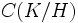# No common composition factor with quotient group is transitive

This article gives the statement, and possibly proof, of a subgroup property (i.e., normal subgroup having no common composition factor with its quotient group) satisfying a subgroup metaproperty (i.e., transitive subgroup property)
View all subgroup metaproperty satisfactions | View all subgroup metaproperty dissatisfactions |Get help on looking up metaproperty (dis)satisfactions for subgroup properties
Get more facts about normal subgroup having no common composition factor with its quotient group |Get facts that use property satisfaction of normal subgroup having no common composition factor with its quotient group | Get facts that use property satisfaction of normal subgroup having no common composition factor with its quotient group|Get more facts about transitive subgroup property

## Statement

Suppose$G$ is a group of finite composition length, and$H \le K \le G$ are subgroups such that$K$ is a normal subgroup of$G$ having no common composition factor with$G/K$ and$H$ is a normal subgroup of$K$ having no common composition factor with$K/H$.

Then,$H$ is a normal subgroup of$G$ having no common composition factor with$G/H$.

## Proof=

Given:$G$ of finite composition length,$H \le K \le G$ with$H$ normal in$K$ and$K$ normal in$G$,$H$ has no common composition factor with$K/H$ and$K$ has no common composition factor with$G/K$.

To prove:$H$ is normal in$G$ and$H$ has no common composition factors with$G/H$.

Proof:

1.$H$ is normal in$G$: This follows from facts (1) and (2).
2.$H$ has no common composition factor with$G/H$: Let$C(L)$ be the set of composition factors of a group$L$. Then,$C(K) = C(H) \cup C(K/H)$ and$C(G/H) = C(G/K) \cup C(K/H)$. By assumption,$C(H)$ and$C(K/H)$ are disjoint. Also, since$C(H) \subseteq C(K)$, and$C(K)$ and$C(G/K)$ are disjoint,$C(H)$ and$C(G/K)$ are disjoint. Thus,$C(H)$ is disjoint from the union$C(G/H) = C(G/K) \cup C(K/H)$.